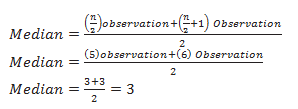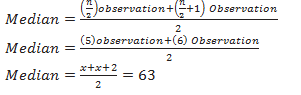# NCERT Solutions Class 9th Maths Statistics

In this page we have NCERT Solutions Class 9th Maths Statistics for EXERCISE 3 . Hope you like them and do not forget to like , social share and comment at the end of the page.

Question 1
The following number of goals was scored by a team in a series of 10 matches:
2, 3, 4, 5, 0, 1, 3, 3, 4, 3
Find the mean, median and mode of these scores.
Solution:
Mean= (Sum of all 10 matches) /10
=(2+3+4+5+0+1+3+3+4+3)/10 =2.8
Median:
We need to arrange the goals in increasing order
0,1,2,3,3,3,3,4,4,5
Since the number of terms is 10 (even)Mode:
We need to arrange the goals in increasing order
0,1,2,3,3,3,3,4,4,5
Since 3 occurs becomes maximum times. It is the mode
Question 2.
In a mathematics test given to 15 students, the following marks (out of 100) are recorded:
41, 39, 48, 52, 46, 62, 54, 40, 96, 52, 98, 40, 42, 52, 60
Find the mean, median and mode of this data.
Solution :
Mean= (Sum of all 15 students) /15
=(41+39+48+52+46+62+54+40+96+52+98+40+42+52+60)/10 =54.8
Median:
We need to arrange the data in increasing order
39,40,40,41,42,46,48,52,52,52,54,60,62,96,98
Since the number of terms is 15(odd)
edian= (n+1)/2=8 term=52
Mode:
52 occurs maximum times.So mode is 52
Question 3.
The following observations have been arranged in ascending order. If the median of the data is 63, find the value of x.
29, 32, 48, 50, x, x + 2, 72, 78, 84, 95
Solution:
The number of term is even
So2x+2=126
x=62

Question 4.
Find the mode of 14, 25, 14, 28, 18, 17, 18, 14, 23, 22, 14, 18.
Solution:
14 occurs maximum time,so mode is 14
Question 5.
Find the mean salary of 60 workers of a factory from the following table
 Salary( in Rs) Number of Workers 3000 16 4000 12 5000 10 6000 8 7000 6 8000 4 9000 3 10000 1 Total 60
Solution
 x f fx 3000 16 48000 4000 12 48000 5000 10 50000 6000 8 48000 7000 6 42000 8000 4 32000 9000 3 27000 10000 1 10000 sum 60 305000
Mean = fx/f= 305000/60=5083.33